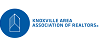#### 2 Listings

{"action":"https:\/\/realestate.windriverliving.com\/idx\/results\/listings","hiddenInputs":"<input name=\"start\" type=\"hidden\" value=\"1\" \/>\n<input name=\"tb\" type=\"hidden\" value=\"\" \/>\n<input name=\"bd\" type=\"hidden\" value=\"\" \/>\n<input name=\"hp\" type=\"hidden\" value=\"5000000\" \/>\n<input name=\"lp\" type=\"hidden\" value=\"0\" \/>\n<input name=\"pt\" type=\"hidden\" value=\"1\" \/>\n<input name=\"idxID\" type=\"hidden\" value=\"c049\" \/>\n<input name=\"acres\" type=\"hidden\" value=\"\" \/>\n<input name=\"sqft\" type=\"hidden\" value=\"\" \/>\n<input name=\"ccz\" type=\"hidden\" value=\"city\" \/>\n<input name=\"fl\" type=\"hidden\" value=\"y\" \/>\n<input name=\"srt\" type=\"hidden\" value=\"prd\" \/>\n<input name=\"csv_listingID\" type=\"hidden\" value=\"\" \/>\n<input name=\"a_streetName\" type=\"hidden\" value=\"\" \/>\n<input name=\"amin_sqFt\" type=\"hidden\" value=\"\" \/>\n<input name=\"amax_sqFt\" type=\"hidden\" value=\"\" \/>\n","maxPerPage":"0","per":"10","currentPage":1,"lastPage":1,"path":"https:\/\/realestate.windriverliving.com\/idx\/results\/listings?start=1&tb=&bd=&hp=5000000&lp=0&pt=1&idxID=c049&per=10&acres=&sqft=&ccz=city&fl=y&srt=prd&csv_listingID=&a_streetName=&amin_sqFt=&amax_sqFt="}© 2023 Knoxville Area Association of Realtors Multiple Listing Association. All rights reserved. Information is provided exclusively for consumers' personal, non-commercial use, and may not be used for any purpose other than to identify prospective properties consumers may be interested in purchasing. Information deemed to be reliable but not guaranteed.
SaveNew SearchModify
Data services provided by IDX Broker